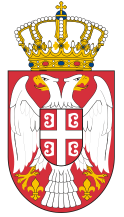Dokument se učitava

# Power Transformers and GeneratorsCourse code: 120707 | 6 ECTS credits

Basic information
Level of Studies: Undergraduate applied studies
Year of Study: 2
Semester: 4
Requirements: None
Goal: The goal of the course is to educate students about operating principles, design, theory, and testing op power transformers and synchronous generators.
Outcome: After exam passed, students should be able to do erection, commissioning, testing and maintenance of power transformers and synchronous generators.
Contents of the course
Theoretical instruction:
1. Power transformers: Basic elements of design.Operating principle and basic equations.
2. Heating issues and rated power. Reduction of secondary parameters and electrical quantities to primary winding and vice versa.
3. Losses and power efficiency. Vector diagrams.
4. Equivalent circuit. No-load operation and short-circuit. Voltage changes.
5. Three-phase transformers. Parallel operation of transformers.
6. Voltage regulation. Autotransformers.
7. Design and application of transformers. Exploitation of transformers.
8. Synchronous generators. Introduction. Design.
9. Operating principle. Losses, power efficiency, and rated values.
10. Armature reaction. Vector diagrams of synchronous generators.
11. Per-unit system and parameters of synchronous generators.
12. Paralel operation of synchronous generators.
13. Angle characteristics.
15. Regulation characteristics and excitation systems.
Practical instruction (Problem solving sessions/Lab work/Practical training):
1. Computational and laboratory exercises follow hours of lectures.
Textbooks and References
1. Đukan Vukić: Energetski transformatori i generatori, VIŠER, Beograd, 2012.
2. Đukan Vukić: Energetski transformatori i generatori - zbirka zadataka, VIŠER, Beograd, 2010.
3. Uputstvo za laboratorijske vežbe.
Number of active classes (weekly)
Lectures: 3
Practical classes: 2
Other types of classes: 0
Grading (maximum number of points: 100)
Pre-exam obligations
Points
activities during lectures
10
activities on practial excersises
0
seminary work
0
colloquium
60
Final exam
Points
Written exam
30
Oral exam
0

#### Associate